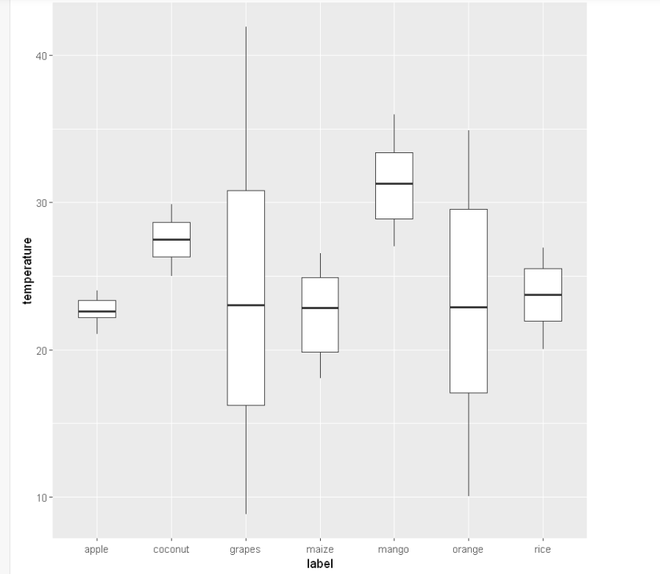Spacing between boxplots in ggplot2 in R

• Last Updated : 18 Jul, 2021

In this article, we are going to see how to add space between the boxplots in ggplot2 using R programming language.

Dataset in use: Crop_recommendation

Method 1: Using width between boxplot

Here we will use width attributes to define space between the boxplot. In this the value is passed to the attribute.

Syntax: geom_boxplot(width)

Program:

R

 library(ggplot2)    # loading data set and storing it in ds variable df <- read.csv("Crop_recommendation.csv", header = TRUE)    # create a boxplot by using geom_boxplot() function # of ggplot2 package plot = ggplot(data=df,               mapping=aes(                 x=label, y=temperature))+ geom_boxplot(width = 0.5) plot

Output:Method 2: Using position_dodge

Here we will use position_dodge to define the vertical position of a geom while adjusting the horizontal position. position_dodge() requires the grouping variable to be specified position.

Syntax:

geom_boxplot( position = position_dodge(width))

Program:

Python3

 library(ggplot2)    # loading data set and storing it in ds variable df <- read.csv("Crop_recommendation.csv", header = TRUE)    # create a boxplot by using geom_boxplot() function # of ggplot2 package plot= ggplot(data=df,              mapping=aes(x=label,                           y=temperature))+ geom_boxplot(width=0.1, position = position_dodge(width=0.5)) plot

Output:My Personal Notes arrow_drop_up
Recommended Articles
Page :